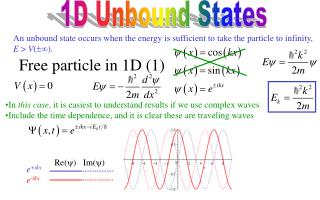Download Presentation1D Unbound States1D Unbound States - PowerPoint PPT Presentation

Download Presentation1D Unbound States
An Image/Link below is provided (as is) to download presentation

Download Policy: Content on the Website is provided to you AS IS for your information and personal use and may not be sold / licensed / shared on other websites without getting consent from its author. While downloading, if for some reason you are not able to download a presentation, the publisher may have deleted the file from their server.

- - - - - - - - - - - - - - - - - - - - - - - - - - - E N D - - - - - - - - - - - - - - - - - - - - - - - - - - -
Presentation Transcript

1. 1D Unbound States Re() Im() e+ikxe-ikx An unbound state occurs when the energy is sufficient to take the particle to infinity, E > V(). Free particle in 1D (1) • In this case, it is easiest to understand results if we use complex waves • Include the time dependence, and it is clear these are traveling waves

2. Re() Im() Free particle in 1D (2) • Comments: • Note we get two independent solutions, and solutions for every energy • Normalization is a problem • Wave packets solve the problem of normalization • The general solution of the time-dependant Schrödinger equation is a linear combination of many waves • Since k takes on continuous values, the sum becomes an integral Wave packets are a pain, so we’ll use waves and ignore normalization

3. I II The Step Potential (1) incident transmitted reflected • We want to send a particle in from the left to scatter off of the barrier • Does it continue to the right, or does it reflect? • Solve the equation in each of the regions • Assume E > V0 • Region I • Region II • Most general solution is linear combinations of these • It remains to match boundary conditions • And to think about what we are doing!

4. I II The Step Potential (2) incident transmitted reflected • What do all these terms mean? • Wave A represents the incoming wave from the left • Wave B represents the reflected wave • Wave C represents the transmitted wave • Wave D represents an incoming wave from the right • We should set D = 0 • Boundary conditions: • Function should be continuous at the boundary • Derivative should be continuous at the boundary

5. I II The Step Potential (3) incident transmitted reflected • The barrier both reflects and transmits • What is probability R of reflection? • The transmission probabilityis trickier to calculate because the speed changes • Can use group velocity, wave packets,probability current • Or do it the easy way:

6. I II The Step Potential (4) incident reflected evanescent • What if V0 > E? • Region I same as before • Region II: we have • Don’t want it to blow up at infinity, so e-x • Take linear combination of all of these • Match waves and their derivative at boundary • Calculate the reflection probability

7. I II T R The Step Potential (5) incident reflected evanescent • When V0 > E, wave totally reflects • But penetrates a little bit! • Reflection probability is non-zero unless V0 = 0 • Even when V0< 0!

8. Sample Problem Electrons are incident on a step potential V0 = - 12.3 eV. Exactly ¼ of the electrons are reflected. What is the velocity of the electrons? • Must have E > 0

9. The Barrier Potential (1) • Assume V0 > E • Solve in three regions I II III • Let’s find transmission probability • Match wave function and derivative at both boundaries • Work, work . . .

10. The Barrier Potential (2) I II III • Solve for : • Assume a thick barrier: L large • Exponentials beat everything

11. Sample Problem An electron with 10.0 eV of kinetic energy is trying to leap across a barrier of V0 = 20.0 eV that is 0.20 nm wide. What is the barrier penetration probability?

12. The Scanning Tunneling Microscope • Electrons jump a tiny barrier between the tip and the sample • Barrier penetration is very sensitive to distance • Distance is adjusted to keep current constant • Tip is dragged around • Height of surface is then mapped out